﻿ 图像边缘检测的量子算法

# 图像边缘检测的量子算法Quantum Algorithm for Edge Detection

Abstract: Edge detection is one of the fundamental problems in the image processing. Many algorithms of edge detection are based on the classical image processing technology. In this paper, we introduce the quantum algorithm of edge detection based on quantum mechanics and its application in image detection. Quantum algorithm is based on the quantization of the classical information of the image and one-to-one correspondence between various elements of the image processing and the concept and variables of quantum mechanics. By constructing the Hamiltonian for the image features, the quantum evolution technology for the quantum information of images learns the profile of the image, so as to give the desired image contour. We use the techniques of the pixel gray gradient, second order difference of pixel gray, Sobel operator convolution as the feature vectors to construct Hamiltonian. Our quantum algorithms are tested by a set of standard pictures (coming from BSDS500 exposed database). The testing results show that our quantum algorithm has a 25% higher sensitivity than the traditional Canny algorithm and has a stronger denoise ability.

1. 引言

2. 经典信息与量子信息对应

2.1. 布洛赫球和量子比特

$|\psi 〉=\alpha |0〉+\beta |1〉$ (1)

${|\alpha |}^{2}+{|\beta |}^{2}=1$ (2)

$|\psi 〉=\mathrm{cos}\theta |0〉+{\text{e}}^{i\varphi }\mathrm{sin}\theta |1〉$ (3)

2.2. 经典边缘检测与量子力学概念的类比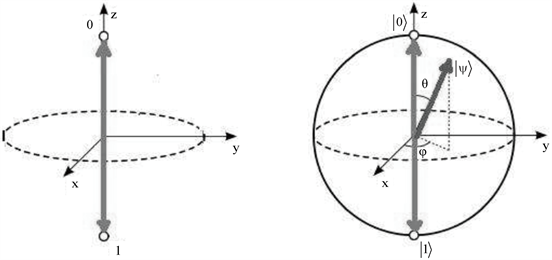Figure 1. Bloch sphere of classical bits and qubitsFigure 2. The analogy between edge detection and quantum mechanics of a single project

2.3. 薛定谔方程的解(4)

(1) 哈密顿量不依赖时间，演化算符可以表示为

$U\left(t,{t}_{0}\right)={\text{e}}^{-\frac{i}{\hslash }\left(t-{t}_{0}\right)H}$ (5)

$|\psi \left(t\right)〉={\text{e}}^{-\frac{i}{\hslash }tH}|\psi \left({t}_{0}\right)〉$ (6)

(2) 哈密顿量与时间有关，不同时间的算符H是可交换的，即

$\left[H\left({t}_{1}\right),H\left({t}_{2}\right)\right]=H\left({t}_{1}\right)H\left({t}_{2}\right)-H\left({t}_{2}\right)H\left({t}_{1}\right)=0$ (7)

$U\left(t,{t}_{0}\right)={\text{e}}^{-\frac{i}{\hslash }{\int }_{{t}_{0}}^{t}H\left(\tau \right)\text{d}\tau }$ (8)

$|\psi \left(t\right)〉={\text{e}}^{-\frac{i}{\hslash }{\int }_{0}^{t}H\left(\tau \right)\text{d}\tau }|\psi \left(0\right)〉$ (9)

(3) 哈密顿量与时间有关，但不同时间的算符H是不可交换的，即

$\left[H\left({t}_{1}\right),H\left({t}_{2}\right)\right]=H\left({t}_{1}\right)H\left({t}_{2}\right)-H\left({t}_{2}\right)H\left({t}_{1}\right)\ne 0$ (10)

3. 量子力学的构造

$H\left(t\right)=g\left(t\right)\stackrel{¯}{S}$ (11)

$\left[H\left({t}_{1}\right),H\left({t}_{2}\right)\right]=g\left({t}_{1}\right)\stackrel{¯}{S}g\left({t}_{2}\right)\stackrel{¯}{S}-g\left({t}_{2}\right)\stackrel{¯}{S}g\left({t}_{1}\right)\stackrel{¯}{S}=0$ (12)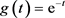(13)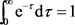(14)

$H=2i\hslash \pi {\text{e}}^{-t}\left(\begin{array}{cc}0& -1\\ 1& 0\end{array}\right)$ (15)

$H=\frac{1}{2}i\hslash \pi {\text{e}}^{-t}\left(\begin{array}{cc}0& -1\\ 1& 0\end{array}\right)$ (16)

$H=i\hslash \pi f\left(\stackrel{¯}{x}\right)\left(\begin{array}{cc}0& -1\\ 1& 0\end{array}\right)$ (17)

$f\left(x\right)=\underset{n=0}{\overset{m}{\sum }}{a}_{n}{x}_{n}^{n}\left(m\in {Z}_{+}\right)$$f\left(x\right)=\underset{n=0}{\overset{m}{\sum }}{a}_{n}{x}^{n}\left(m\in {Z}_{+}\right)$$f\left(x\right)={W}_{2}\cdot \frac{1}{1+{\text{e}}^{-{W}_{1}\cdot X+{b}_{1}}}+{b}_{2}$ (18)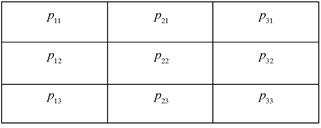Figure 3. Pixel arrangement and four-direction gray difference extraction

$f\left(\stackrel{¯}{x}\right)={a}_{0}+{a}_{1}{x}_{1}+{a}_{2}{x}_{2}^{2}+{a}_{3}{x}_{3}^{3}+{a}_{4}{x}_{4}^{4}$ (19)

${e}_{ij}=\left\{\begin{array}{cc}1& {p}_{ij}^{train}\ne {p}_{ij}^{window}\\ 0& {p}_{ij}^{train}={p}_{ij}^{window}\end{array}$ (20)

$\sum {e}_{ij}$ 训练误差值取最小值时， $f\left(\stackrel{¯}{x}\right)$ 的系数 ${a}_{n}$ 训练成功。

4. 核心算法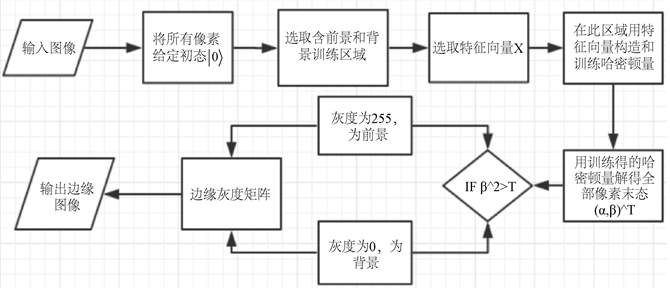Figure 4. Basic flow chart of quantum algorithm

5. 测试结果与分析

5.1. 实验准备

$\text{Sensitivity}=\frac{\text{TP}}{\text{TP}+\text{FN}}$ (21)

$\text{Specificity}=\frac{\text{TN}}{\text{TN}+\text{FP}}$ (22)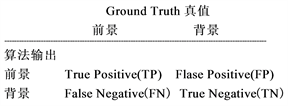Figure 5. Confusion matrix for performance evaluation

5.2. 测试结果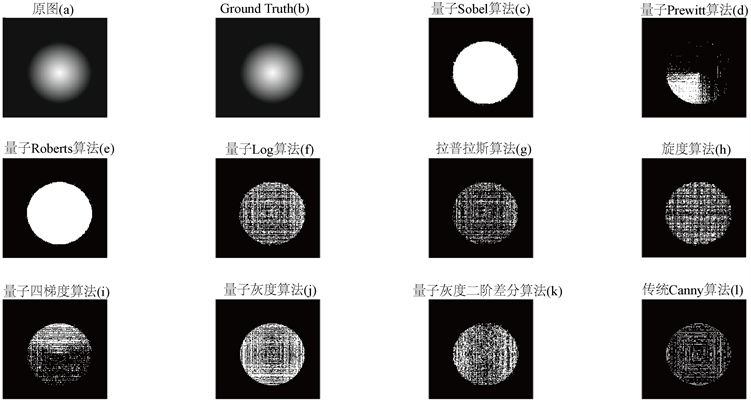Figure 6. Edge Detection of an image with a gradientTable 1. Test results of nine quantum edge detection algorithms and Canny edge detection algorithms

5.3. 分析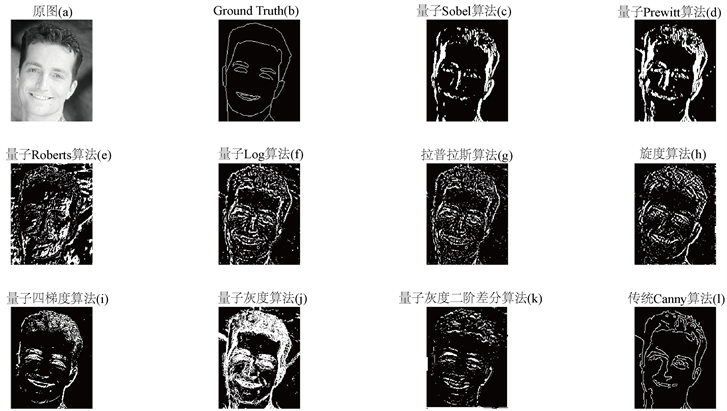Figure 7. Edge Detection of a face image from BSDS500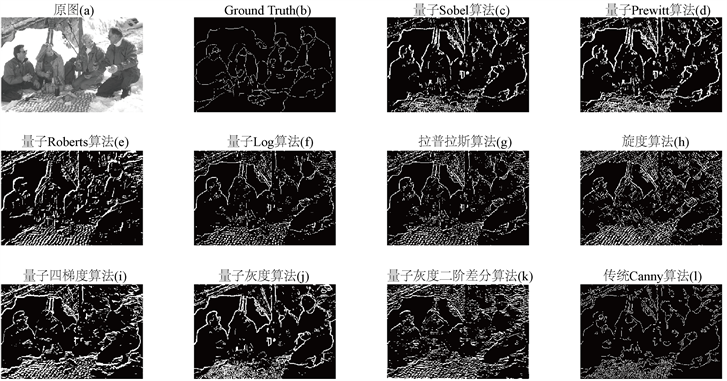Figure 8. Edge Detection of a people image from BSDS500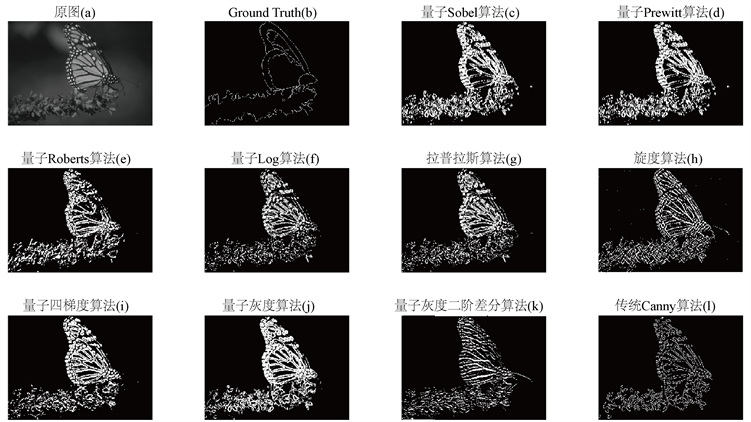Figure 9. Edge Detection of a butterfly image from BSDS500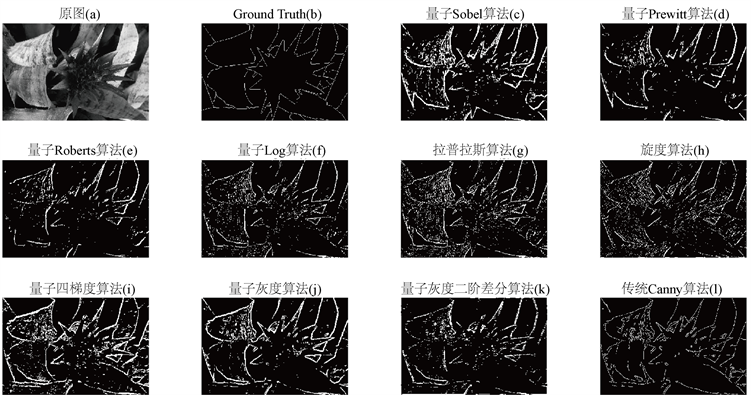Figure 10. Edge Detection of a plant image from BSDS500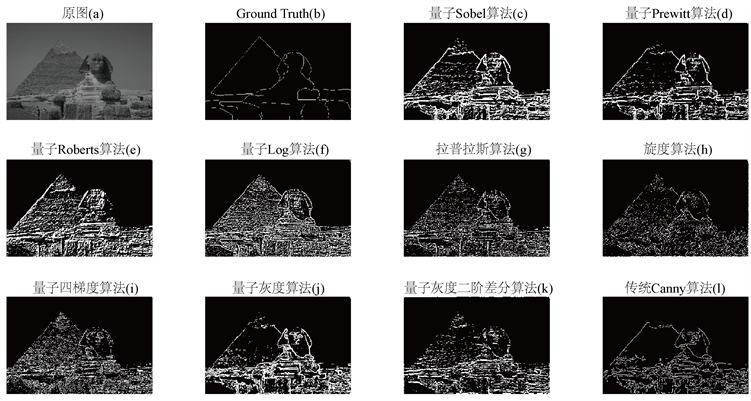Figure 11. Edge Detection of a pyramid image from BSDS500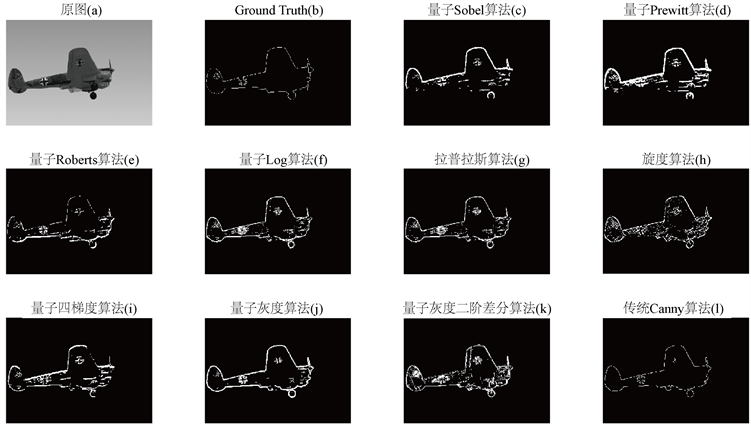Figure 12. Edge Detection of a plane image from BSDS500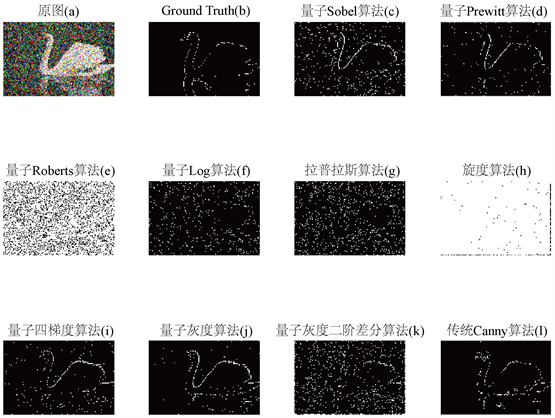Figure 13. Edge Detection of a swam image with noise from BSDS500

6. 总结与展望

NOTES

*通讯作者。

 Marr, D. and Hildreth, E. (1980) Theory of Edge Detection. Proceedings of the Royal Society of London, London, 187-217.
https://doi.org/10.1098/rspb.1980.0020

 Canny, J. (1986) A Computational Approach to Edge Detection. IEEE Transactions on Pattern Analysis and Machine Intelligence, 6, 679-698.
https://doi.org/10.1109/TPAMI.1986.4767851

 Huang, J.S. and Tseng, D.H. (1988) Statistical Theory of Edge Detection. Computer Vision Graphics and Image Processing, 43, 337-346.
https://doi.org/10.1016/0734-189X(88)90087-4

 Zhao, J.-Y., Wang, H.-M. and Yu, D.-H. (2003) A New Approach for Edge Detection of Noisy Image Based on CNN. International Journal of Circuit Theory and Applications, 31, 119-131.
https://doi.org/10.1002/cta.210

 Tian, H.-P. (2011) A Survey of Image Edge Detection Based on GreyTheory. Advanced Materials Research, 301-303, 853-858.
https://doi.org/10.4028/www.scientific.net/AMR.301-303.853

 Koenderink, J.J. (2012) Theory of “Edge Detection”. In: Åström, K., Persson, L.E. and Silvestrov, S., Eds., Analysis for Science, Engineering and Beyond, Springer Proceedings in Mathematics, Springer, Berlin, 35-49.
https://doi.org/10.1007/978-3-642-20236-0_3

 Eldar, Y. and Oppenheim, A.V. (2002) Quantum Signal Processing. IEEE Signal Processing Magazine, 19, 12-32.
https://doi.org/10.1109/MSP.2002.1043298

 Aytekin, C., Kiranyaz, S. and Gabbouj, M. (2013) Quantum Mechanics in Computer Vision: Automatic Object Extraction. 2013 IEEE International Conference on Image Processing, Melbourne, 15-18 September 2013, 2489-2493
https://doi.org/10.1109/ICIP.2013.6738513

 Fu, X.-W., Ding, M.-Y., Sun, Y.-G. and Chen, S.-B. (2009) A New Quantum Edge Detection Algorithm for Medical Images. Proceedings of SPIE—The International Society for Optical Engineering, 7497, Article ID: 749724.
https://doi.org/10.1117/12.832499

 Lou, L.-T., Lu, L., Li, L.-G., Gao, W.-L., Li, L.-L. and Fu, Z.-L. (2009) Automatic contour extraction for Multiple Objects Based on Schroedinger Transform of Image. Proceedings of SPIE—The International Society for Optical Engineering, 7495, Article ID: 749545.
https://doi.org/10.1117/12.829020

 Youssry, A., El-Rafei, A. and Elramly, S. (2015) A Quantum Mechanics-Based Framework for Image Processing and Its Application to Image Segmentation. Quantum Information Processing, 14, 3613-3638.
https://doi.org/10.1007/s11128-015-1072-3

 Mastriani, M. (2014) Quantum Edge Detection for Image Segmentation in Optical Environments.
https://arxiv.org/abs/1409.2918

 Mastriani, M. (2014) Optimal Estimation of States in Quantum Image Processing. http://export.arxiv.org/abs/1406.5121

 Mastriani, M. (2014) Quantum Image Processing? http://export.arxiv.org/abs/1512.02942

 Yuan, S.-Z., Venegas-Andraca, S.E., Wang, Y.-C., Luo, Y. and Mao, X.-F. (2019) Quantum Image Edge Detection Algorithm. International Journal of Theoretical Physics, 58, 2823-2833.
https://doi.org/10.1007/s10773-019-04166-9

 Butkovskiy, A.G. and Samoilenko, Y.I. (1990) Control of Quantum-Mechanical Processes and System. Springer, Berlin.
https://doi.org/10.1007/978-94-009-1994-5

 Arbelaez, P., Maire, M., Fowlkes, C. and Malik, J. (2011) Contour Detection and Hierarchical Image Segmentation. IEEE Transactions on Pattern Analysis & Machine Intelligence, 33, 898-916.
https://doi.org/10.1109/TPAMI.2010.161

 University of California, Berkeley. Berkley Segmentation Data Set and Benchmarks 500.
https://www2.eecs.berkeley.edu/Research/Projects/CS/vision/grouping/resources.html

 Nielsen, M. and Chuang, I. (2007) Quantum Computation and Quantum Information. Cambridge University Press, Cambridge.

 Zhang, Y., Lu, K. and Gao, Y.H. (2015) QSobel: A Novel Quantum Image Edge Extraction Algorithm. Science China Information Sciences, 58, 1-13.
https://doi.org/10.1007/s11432-014-5158-9

Top